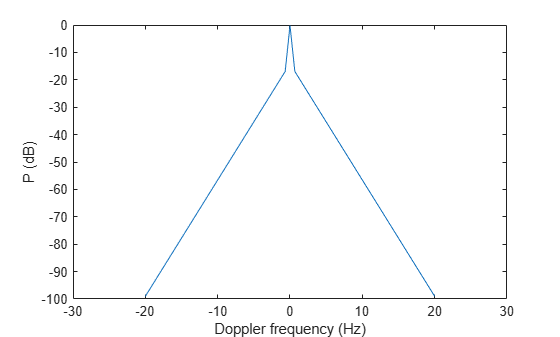# billingsleyicm

Billingsley’s intrinsic clutter motion (ICM) model

## Syntax

``P = billingsleyicm(fd,fc,wspeed)``
``P = billingsleyicm(fd,fc,wspeed,c)``

## Description

example

````P = billingsleyicm(fd,fc,wspeed)` calculates the clutter Doppler spectrum shape, `P`, due to intrinsic clutter motion (ICM) at Doppler frequencies specified in `fd`. ICM arises when wind blows on vegetation or other clutter sources. This function uses Billingsley’s model in the calculation. `fc` is the operating frequency of the system. `wspeed` is the wind speed.```
````P = billingsleyicm(fd,fc,wspeed,c)` specifies the propagation speed `c` in meters per second.```

## Examples

collapse all

Calculate and plot the Doppler spectrum shape predicted by the Billingsley ICM model. Assume the PRF is 2 kHz, the operating frequency is 1 GHz, and the wind speed is 5 m/s.

```v = -3:0.1:3; fc = 1e9; wspeed = 5; c = physconst('LightSpeed'); fd = 2*v/(c/fc); p = billingsleyicm(fd,fc,wspeed); plot(fd,pow2db(p)) xlabel('Doppler frequency (Hz)') ylabel('P (dB)')```## Input Arguments

collapse all

Doppler frequencies in hertz, specified as a scalar or a vector.

Data Types: `double`

Operating frequency of the system in hertz, specified as a scalar.

Data Types: `double`

Wind speed in meters per second, specified as a scalar.

Data Types: `double`

Propagation speed in meters per second, specified as a positive scalar.

Example: `343` meters per second approximates the speed of sound at sea level and at a temperature of 20 °C under normal atmospheric conditions.

Data Types: `double`

## Output Arguments

collapse all

Shape of the clutter Doppler spectrum due to intrinsic clutter motion, returned as a scalar or vector. `P` is the same size as `fd`.

 Billingsley, J. Low Angle Radar Clutter. Norwich, NY: William Andrew Publishing, 2002.

 Long, Maurice W. Radar Reflectivity of Land and Sea, 3rd Ed. Boston: Artech House, 2001.